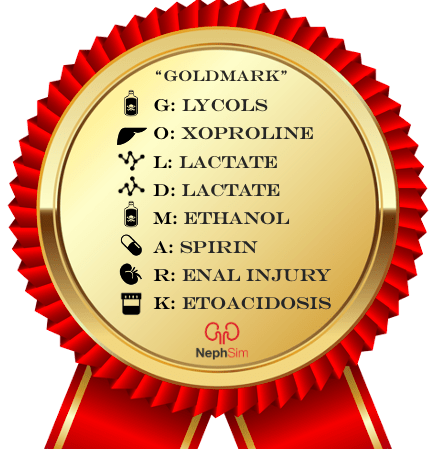# Acid/Base

A trick to solving every acid/base problem:  pLACO

1. pH – The normal range for arterial blood pH is 7.35 – 7.45.
• Looking at the pH will help identify the primary acid/base disturbance
• < 7.35 = acidemia
• >7.45 = alkalemia
• Several studies have shown that venous blood pH can estimate arterial pH by adding approximately 0.04 to the venous pH (normal range for venous pH is appr. 7.31 – 7.41). Note: this estimation does not hold if the patient is in shock.

2. “Labs”: PCO2 & HCO3 – Looking at both the PCO2 and HCO3 will identify the primary process that led to the pH change. Arterial PCO2 can be estimated by subtracting 4 mm Hg from the venous PCO2.
• For an acidemia: If the PCO2 is elevated PCO2 (>45) , the primary process is a respiratory acidosis. If the HCO3 is low (<22), the primary process is a metabolic acidosis.
• For an alkalemia: If the PCO2 is decreased PCO2 (<35) , the primary process is a respiratory alkalosis. If the HCO3 is high (>32), the primary process is a metabolic alkalosis.

3. Calculate the anion gap (AG) – Use the bicarbonate from the chemistry panel to calculate the AG, or unmeasured anions (AG = Na – Cl – HCO3). To identify an elevated anion gap, estimate the patient’s expected or normal anion gap by using the serum albumin. Lower albumin levels lead to a lower normal anion gap, as albumin is an anion. There are different ways of estimating this…
• Try this: albumin x 2.5 = patient’s expected AG (e.g. if the albumin is 2, the anion gap should be 5 – so a calculated AG of 10 is abnormal)
• The anion gap should be checked even in the absence of an acidemia as a metabolic acidosis may be hiding!
• If the anion gap is elevated, think GOLDMARK as you develop your differential diagnosis4. Compensation – Once the primary process has been identified, use the formulas below to see if the patient is compensating. If compensation is inadequate, then there may be another process going on! (i.e. if a patient’s expected pCO2 is lower than the calculated compensation, there is a concomitant respiratory alkalosis). The body will never overcompensate for a primary process, and even with compensation the pH may not return to normal. Respiratory compensation is almost immediate while metabolic (renal) compensation may take several days.
• Metabolic acidosis: compensated PCO2 (within 2 mm Hg) = 1.5 (HCO3) + 8 (Winter’s formula)
• Metabolic alkalosis: For every 1 meq/L increase in HCO3, PCO2 should increase by 0.7 mm Hg
• Acute respiratory acidosis (RAc): for every 10 mmHg increase in the PCO2, there should be a 1 meq/L increase in HCO3
• Chronic RAc: for every 10 mmHg increase in the PCO2, there should be a 4 meq/L increase HCO3
• Acute respiratory alkalosis (RAl): for every 10 mmHg decrease in the PCO2, there should be a 2 meq/L decrease HCO3
• Chronic RAl: for every 10 mmHg decrease in the PCO2, there should be a 4 meq/L decrease HCO3

5. Other processes (do this step only if there is an AG metabolic acidosis)  – A pt can have up to 3 acid-base disorders – an anion gap metabolic acidosis may coexist with a metabolic alkalosis OR non-gap metabolic acidosis AND 1 respiratory disorder (you can only hyperventilate or hypoventilate).
To uncover this process, use the Δ/Δ which will tell you if the change (or Δ) in the HCO3 is fully accounted for by the change in the anion gap. If it isn’t, then another process is driving the HCO3 up (metabolic alkalosis) or down (metabolic acidosis). There are different methods of approaching this, but one method is:
• Δ/Δ = (Anion gap – normal anion gap) / (Pt’s HCO3 – normal HCO3)
• If the ratio above is > 2, then there is a concomitant metabolic alkalosis. If it is < 1, then there is a concomitant non-anion gap metabolic acidosis (NAGMA).
The O also stands for osmolality – remember to compare the serum osmolality (osm) with the calculated osm which =  2*Na + BUN/2.8 + glucose/18 (both glucose and BUN should be in mg/dL for this calculation). If there is a difference of more than 15, think about what the additional osm might be, most often an alcohol (isopropyl alcohol, ethanol, methanol, propylene glycol).

Side note:  If you suspect a non-gap metabolic acidosis, check a “urine anion gap”  (UAG) to differentiate between GI and renal losses of HCO3. If the number is negative (think neGUTive), HCO3 is being lost from the gut or GI tract (diarrhea/fistula). If it’s positive, HCO3 is being lost renally. UAG = urine Na + urine K – urine Cl

Acid-Base Tweetorial: